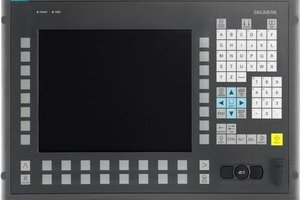# SINUMERIK 840D Programming WHILE Loop UsageSINUMERIK 840D Programming

This blog post is about sinumerik 840D cnc control from Siemens. This post illustrates one of the loop commands (control structures), which we can use in cnc  programming for sinumerik 840D cnc control.

There are multiple control structures which can be used in Sinumerik 840D programming like

• IF
• LOOP
• FOR
• WHILE
• REPEAT
Here is the cnc programming example which shows the usage of the WHILE loop.

#### CNC Program Example

```G01 G90 X0 Z10 F1000
WHILE \$AA_IM[X] <= 100
G1 G91 X10 F500
G1 G90 Z–20 F100
Z5
ENDWHILE```

#### Explanation Of CNC Program

This cnc program cuts a slot (can be used for drilling) on the component face. The cnc program starts from x0 and goes till x100 every time taking a cut in x-axis of 10.

G01 : Linear Interpolation
G90 : Absolute Programming
G91 : Incremental Programming

The first cnc program block takes tool to starting position. Which is x-axis x0 and z-axis z10.

The second cnc program block checks if the x-axis current position is less then or is equal to 100 with sinumerik 840D system variable, and start a WHILE loop.

Then tool travel is x-axis 10 from the current position. (Because G91 Incremental Programming is active, So tool will travel as taking the current tool position as the reference point (or start point).)

Then our tool travel -20 in z-axis (Now G90 Absolute Programming is active so tool will travel with reference to the work piece zero point.)

Now again the tool will be back at z5

The ENDWHILE ends the WHILE loop

So the program will be repeated again and again until the statement which is given with WHILE becomes FALSE.